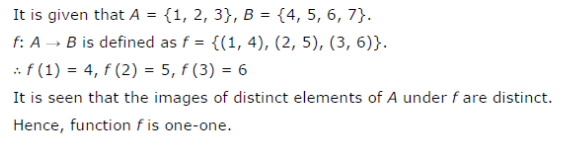# Let A = {1, 2, 3}, B = {4, 5, 6, 7} and let f(x) = {(1, 4), (2, 5), (3, 6)} be a function from A to B

Let A = {1, 2, 3}, B = {4, 5, 6, 7} and let f(x) = {(1, 4), (2, 5), (3, 6)} be a function from A to B. Show that f is one-one.